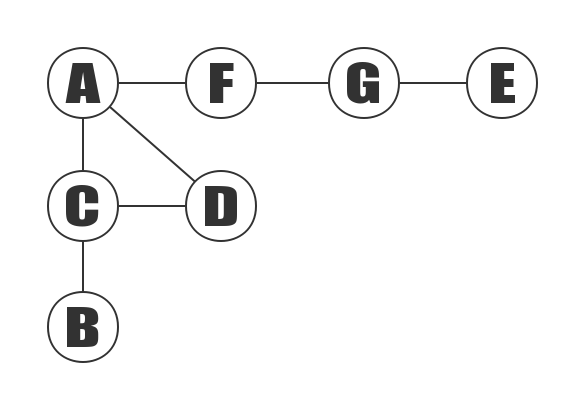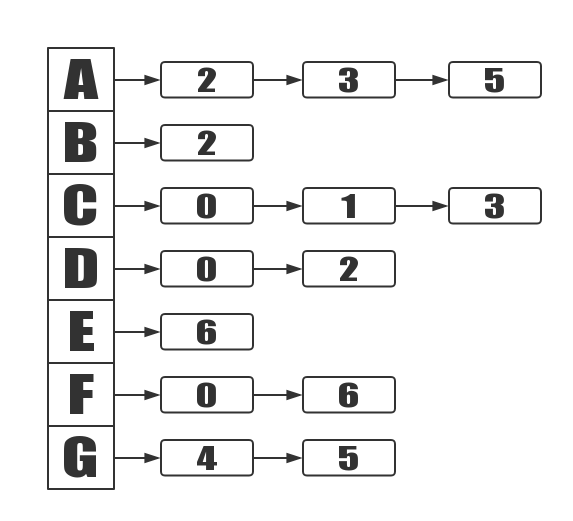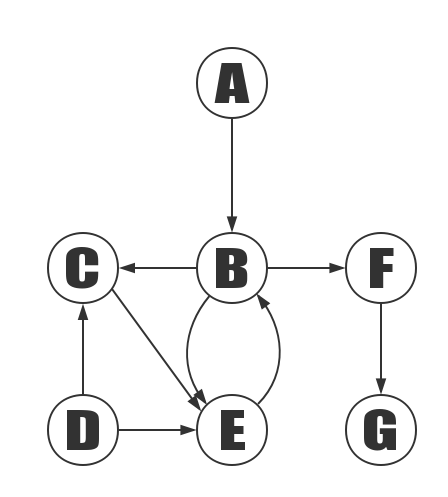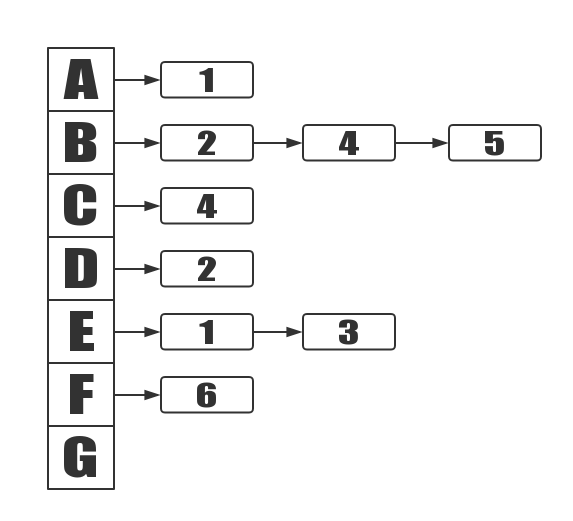Adjacency matrix undirected graph refers to an undirected graph represented by an adjacency list.The above graph contains 7 vertices of A, B, C, D, E, F, G, and it also contains<A,C>, <A,D>, <A,F>, <B,C>, <C,D>, <E,G>, <F,G>, in total 7 edges. Since this is an undirected graph, the edge <A,C> and the edge <C,A> are the same edges. The table of edges is listed in alphabetical order..Each vertex contains a linked list that records the index of vertexs. For example, the data of the nodes included in the linked list included in the second vertex (vertex C) is 0, 1, 3, and 0, 1, 3 corresponds to the index of A, B, D.

### C++ Implementation

Adjacency matrix directed graph refers to an directed graph represented by an adjacency list.The above graph contains 7 vertices of A, B, C, D, E, F, G, and it also contains <A,B>, <B,C>, <B,E>, <B,F>, <C,E>, <D,C>, <E,B>, <E,D>, <F,G>, in total 7 edges.Each vertex contains a linked list that records the index of vertexs.

The linked list contains the index of the other vertex of the out-edge corresponding to this vertex. For example, the data of the first vertex (vertex B) in list is 2,4,5, this 2,4,5 corresponds to index of C, E, F, and C, E, F are the other vertices of B vertex’s out-edge.

Share